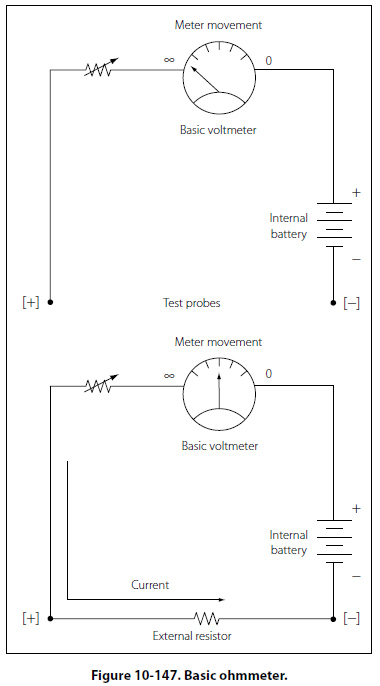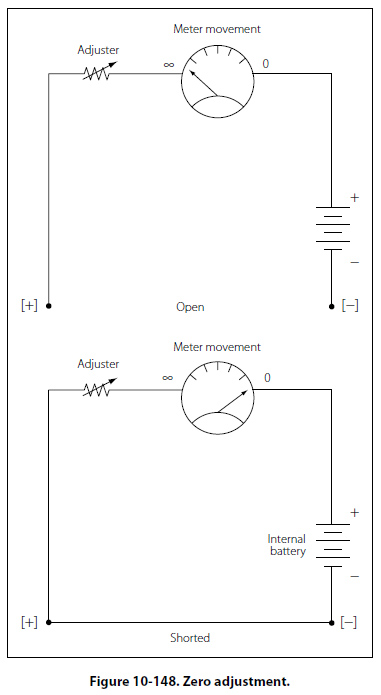Voltmeter Circuit Connections When voltmeters are used, they are connected in parallel with a circuit. If unsure about the voltage to be measured, take the first reading at the high value on the meter and then progressively move down through the range until a suitable read is obtained. Observe that the polarity is correct before connecting the meter to the circuit or damage will occur by driving the movement backwards. Influence of the Voltmeter in the Circuit When a voltmeter is connected across two points in a circuit, current will be shunted. If the voltmeter has low resistance, it will draw off a significant amount of current. This will lower the effective resistance of the circuit and change the voltage readings. When making a voltage measurement, use a high resistance voltmeter to prevent shunting of the circuit. The Ohmmeter The meter movement used for the ammeter and the voltmeter can also be used for the ohmmeter. The function of the ohmmeter is to measure resistance. A simplified one-stage ohmmeter is illustrated in Figure 10-147, which shows that the basic ohmmeter contains a battery and a variable resistor in series with the meter movement. To measure resistance, the leads of the meter are connected across an external resistance,which is to be measured. By doing this the ohmmeter circuit is completed. This connection allows the internal battery to produce a current through the movement coil, causing a deflection of the pointer proportional to the value of the external resistance being measured. Zero Adjustment When the ohmmeter leads are open as shown in Figure 10-148, the meter is at a full scale deflection, indicating an infinite (8) resistance or an open circuit. When the leads are shorted as shown in figure “zero adjust," the pointer will be at the full right hand position, indicating a short circuit or zero resistance. The purpose of the variable resistor in this figure is to adjust the current so that the pointer is at exactly zero when the leads are shorted. This is used to compensate for changes in the internal battery voltage due to aging.©AvStop Online Magazine                                                                                                                                                      Contact Us              Return To Books